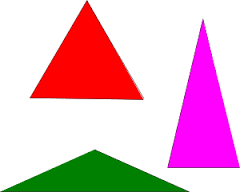# Isosceles triangle

Find the area of an isosceles triangle whose leg is twice the base, b=1

A =  0.9682

### Step-by-step explanation:

Try calculation via our triangle calculator.Did you find an error or inaccuracy? Feel free to write us. Thank you!

Tips for related online calculators
Need help calculating sum, simplifying, or multiplying fractions? Try our fraction calculator.
Calculation of an isosceles triangle.
Do you want to convert time units like minutes to seconds?